A radical equation is an equation in which a variable is under a radical. To solve a radical equation:

1. Isolate the radical expression involving the variable. If more than one radical expression involves the variable, then isolate one of them.

2. Raise both sides of the equation to the index of the radical.

3. If there is still a radical equation, repeat steps 1 and 2; otherwise, solve the resulting equation and check the answer in the original equation.

By raising both sides of an equation to a power, some solutions may have been introduced that do not make the original equation true. These solutions are called extraneous solutions.

##### Example 1

Solve.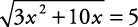Raise both sides to the index of the radical; in this case, square both sides.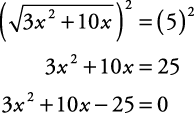This quadratic equation now can be solved either by factoring or by applying the quadratic formula.

Applying the quadratic formula,Now, check the results.

If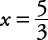,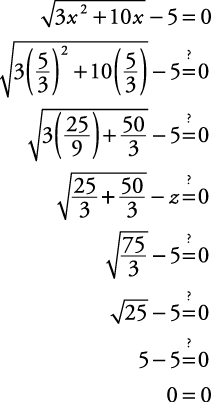If x = –5,The solution isor x = –5.

##### Example 2

Solve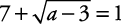.There is no solution, sincecannot have a negative value.

##### Example 3

Solve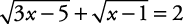.

Isolate one of the radical expressions.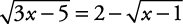Raise both sides to the index of the radical; in this case, square both sides.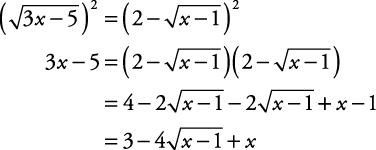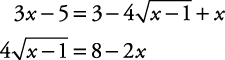Raise both sides to the index of the radical; in this case, square both sides.This can be solved either by factoring or by applying the quadratic formula.

Applying the quadratic formula,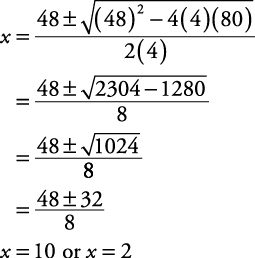Check the solutions.

If x = 10,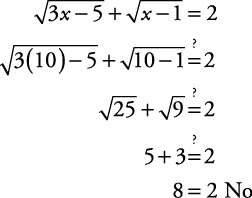So x = 10 is not a solution.

If x = 2,The only solution is x = 2.

##### Example 4

Solve.

Isolate the radical involving the variable.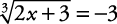Since radicals with odd indexes can have negative answers, this problem does have solutions. Raise both sides of the equation to the index of the radical; in this case, cube both sides.The check of the solution x = –15 is left to you.